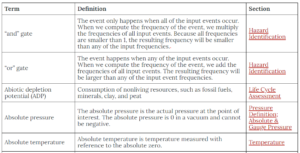# How to Use This Book

## If you find any mistakes in this book, please fill out the survey in this link, or contact Dr. Jonathan Verrett at jonathan.verrett@ubc.ca.

Pressbook can be exported in various types of files. However, the codes to show mathematical equations are not compiled in some of the file types. We recommend viewing this textbook online because it shows the best format for displaying mathematical equations.

# Chapters

This book is divided into 8 chapters. Once you select a chapter, there will be a short introduction text along with the chapter’s learning objectives. The learning objects can help guide your learning as you go through each chapter. Here is an example:

## Introduction

In this chapter, we will introduce some of the basics in the design aspect of chemical and biological engineering. We will focus on basic engineering and general engineering economics. This chapter also introduces the components and characteristics of 4 key process diagrams.

Learning Objectives

By the end of this chapter, you should be able to:

Define the components of a design basis memorandum

Apply basic measures of economic merit to perform initial estimates of project economic feasibility

Recognize the 4 key types of flow sheets

Create input-output diagrams

Construct block flow diagrams (BFDs) for chemical processes

Define common unit operations and use these when constructing process flow sheets

Recognize the need to apply basic material balances

Build process flow diagrams (PFDs)

Understand piping and instrumentation diagrams (P&IDs)

We’ve also included terms you should take note of or keep in mind while you’re going through the chapter:

As you’re going through this chapter, here are some important terms for you to take note of:

• basic engineering
• basic design package
• design basis memorandum (DBM)
• inside battery limit
• outside battery limit
• gross economic potential (GEP)
• net economic potential (NEP)
• capital costs (CC)
• return of investments (ROI)
• input-output diagram
• block flow diagram (BFD)
• process flow diagram (PFD)
• piping and instrumentation diagram (P&ID)
• unit operation
• mixer
• splitter
• separator
• reactor
• capillary connection
• pneumatic connection
• electrical connection

# Sections

Each chapter has sections focusing on more specific topics within the chapter. Each section in the chapter starts off with the learning objectives that are specific to that section.

Learning Objectives

By the end of this chapter, you should be able to:

### Define the components of a design basis memorandum

In each section, we’ve included 2 types of questions. The first question type is an “Example” (purple text box). These examples are used as an educational guide to demonstrate how problems in this section are approached. You can use these examples to learn how questions are solved step by step. The second question type is an “Exercise” (blue text box). These exercises are made for you to test your knowledge. Try solving the question before looking at the solution to see how much you know and what concepts you should review.

Example

### Production of Vinyl Chloride

Say we want to build a plant that produces 360,000 tonnes per year of a vinyl chloride monomer ($C_{2}H_{3}Cl$) to be used in the production of polyvinyl chloride (PVC). One reaction pathway is shown below.

$C_{2}H_{4} + Cl_{2} → C_{2}H_{4}Cl_{2}\;\;\;\;\; (1)$

$C_{2}H_{4}Cl_{2} → C_{2}H_{3}Cl + HCl\;\;\;\;\; (2)$

A DBM for this process might consist of the following items:

For contractual issues:

• High level description of vinyl chloride and uses
• Ethane and chlorine feeds to be used
• Reactor type being used
• Plant capacity of 360,000 tonnes/yr of vinyl chloride, located in Houston, Texas
• Operating composition purity, temperature, and pressure
• Operating philosphy
• Gantt chart showing project timeline

For site-specific issues:

• Temperature, pressure, and composition of feed (inside battery limits)
• Cooling water used at $25 ^{\circ}C$ and 2 atm and steam at $20^{\circ}C$ and 3 atm (outside battery limits)
• Emission predictions and requirements
• Atmospheric pressure, little wind and rainfall, maximum and minimum temperatures at location
• Environmental and safety issues at locations
• Control and electrical requirements of the plant

For design-specific issues:

• Equipment, instrument, and line numbering
• Units used in calculation (imperial or SI)
• Standards
• Certain technical equipment have certain standards (pressure vessels, heat exchangers, electrical work)

Exercise

### Benzene Production

Consider a process where toluene is fed into a process at 1000 kg/hr and hydrogen is fed at 82 kg/hr. The following reaction takes place in the process:

$C_{7}H_{8} + H_{2} ➡ C_{6}H_{6} + CH_{4}$

The reaction achieves 75% conversion and the products of the process are separated into 2 streams. The first product stream is benzene, exiting at 821 kg/hr. The second product stream is a mixed gas stream (including the unreacted reactants and by-products) exiting at 261 kg/hr. Draw an input-output diagram for this process.

Solution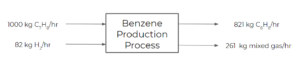In some sections, we have put in extra useful information (such as resources or finding data). We’ve used grey text boxes for these information sources.

### Finding Chemical Pricing Data

Finding this data can be very challenging. Companies typically want to keep pricing information secret (for competition reasons). The best way I have found to search for chemical pricing data is as follows:

1. Go to Texas A&M Chemical Pricing Database
2. Search the chemical by name (i.e. Ethylene) or CAS number (Chemical Abstracts Services; can be found on Wikipedia)
3. Find a citation from ‘ICIS Chemical Business’ and note the edition and page number (i.e. 2018, April 6; 19)
4. Go to UBC Library and type in “ICIS Chemical Business” into the search bar and search
5. Click on the source by Factiva, Business Source Ultimate
6. Click on ‘Browse Journal’
7. Find the citation you found from the Texas A&M Database citation using the dates
8. Once you find your article, click ‘UBC eLink’. This should take you to a new page where you can access the full text
9. Search through the article for the chemical of interest’s price

This process is tedious and often takes some time, even for people experienced in this process. So don’t get discouraged when looking for this data and feel free to ask questions (through the discussion board, in tutorials or office hours, etc.).

# Chapter Reviews

This book has a chapter review section at the end of each chapter that consists of important equations discussed in the chapter as well as a set of flashcards you can use to gauge how well you understand the concepts discussed in the chapters. Note that you don’t need to memorize these terms, but it is important to understand what the terms mean and the context in which they appear.

## Important Equations

 Kinetic Energy $E_{k} = \frac{1}{2} mu^{2}$ $\dot{E}_{k} = \frac{1}{2} \dot{m} u^{2}$ Potential Energy $E_{p} = m g z$ $\dot{E}_{p} = \dot{m} g z$ $\Delta E_{p} = E_{p2} - E_{p1} = m g (z_{2} - z_{1})$ First Law of Thermodynamics $\Delta U + \Delta E_{k} + \Delta E_{p} = Q + W$ Flow Work $\dot{W}_{fl} = \dot{W}_{fl-in} - \dot{W}_{fl-out} = P_{in}\dot{V}_{in} - P_{out}\dot{V}_{out}$ Steady-state Open System Energy Balance $\dot{Q} + \dot{W} = \Sigma_{out} \dot{E}_{j} - \Sigma_{in} \dot{E}_{j}$ $\dot{Q} + \dot{W}_{s} = \Delta\dot{H} + \Delta\dot{E}_{k} + \Delta\dot{E}_{p}$ Enthalpy $\hat{H} = \hat{U} + P\hat{V}$ $\Delta\hat{H} = \Sigma_{i}\Delta\hat{H}_{i}$ Heat Capacity (closed system) $C_{V}(T) = \bigg(\frac{\delta\hat{U}}{\delta T}\bigg)_{V}$ Internal Energy (closed system) $d\hat{U} = C_{V}(T)dT$ $\Delta\hat{U} = \int^{T_{2}}_{T_{1}}C_{V}dT$ Heat Capacity (open system) $C_{P}(T) = \bigg(\frac{\delta\hat{H}}{\delta T}\bigg)_{P}$ Enthalpy (open system) $\Delta\hat{H} = \int^{T_{2}}_{T_{1}}C_{P}dT$ Heat of Reaction Method $\Delta\dot{H} = \xi\Delta\dot{H}_{r} + \Sigma\dot{n}_{out}*\int^{T_{out}}_{T_{ref}}C_{P}dT - \Sigma\dot{n}_{in}*\int^{T_{in}}_{T_{ref}}C_{P}dT$ Heat of Formation Method $\xi\Delta\dot{H}^{\circ}_{r} = \Sigma\dot{n}_{out}*\hat{H}^{\circ}_{f,i} - \Sigma\dot{n}_{in}*\hat{H}^{\circ}_{f,i}$

# Chapter Exercises

A section consisting of extra practice problems is included at the end of each chapter. The purpose of this section is to give you more practice problems to work on as well as get more confident with the course material. The exercises consist of 3 question types: multiple-choice questions, short answer questions, and long answer questions. This allows you to practice solving different question types and timing yourself. The solutions to the questions are posted below the question in a different text box. We would highly recommend solving the problem before looking over the solution.

Exercise (Multiple Choice)

Consider a reactor in which an endothermic reaction takes place and 15.8 kJ are absorbed. A mixer is used in the reactor and provides 6.3 kJ of work on the system. What is the overall heat that needs to be provided to the system?

a) -22.1 kJ

b) 9.5 kJ

c) 22.1 kJ

d) -9.5 kJ

### Solution

b) 9.5 kJ
Performing an energy balance on the system, we know that $\Delta H = Q + W$. Since this is an endothermic reaction and work is done on the system, both the enthalpy of the reaction and the work are positive:
\begin{align*}
Q&= \Delta H -W \\
&= 15.8 kJ – 6.3 kJ\\
&=9.5kJ
\end{align*}

You have a feed stream of methanol at 1 atm and $25^{\circ}C$. You’d like to bring it to a temperature of $100^{\circ}C$ so that it enters a reactor as a gas. The boiling point of methanol is $65^{\circ}C$ at 1 atm. What process path (with enthalpy changes) can you take to achieve this change and calculate the overall enthalpy?

### Solution

The following steps can be taken to calculate the overall change in enthalpy of going from liquid methanol at 1 atm and $25^{\circ}C$ to gas methanol at 1 atm and $100^{\circ}C$.

1. Enthalpy change from $25^{\circ}C$ to $65^{\circ}C$ using the liquid state heat capacity of methanol
2. Heat of vapourization of methanol at a constant temperature of $65^{\circ}C$
3. Enthalpy change from $65^{\circ}C$ to $100^{\circ}C$ using the gas state heat capacity of methanol

The enthalpy change of the process will be the sum of steps 1-3.

Consider the combustion of ethylene:

C2H4 (g) + 3 O2 (g) → 2 CO2 (g) + 2 H2O (g)

The enthalpy of combustion can be determined using the enthalpies of the following reactions:

• Reaction 1: C2H2 + H2 → C2H$\Delta H_{r1} = -174.19 kJ$
• Reaction 2: 2 C2H2 + 5 O2 → 4 CO2 + 2 H2O $\Delta H_{r2} = -2511 kJ$
• Reaction 3: 2 CO2 + H2 → 2 O2 + C2H2 $\Delta H_{r3} = 1013.7 kJ$

Determine the enthalpy of the combustion of ethylene from reactions 1, 2, and 3 using Hess’s Law at standard temperature and pressure.

### Solution

Step 1: Determine the combination of reactions that will give us the desired combustion reaction.

We know that we need C2H4 in the reactants, so we multiply reaction 1 by -1. We notice that by adding the other 2 reactions to reaction 1 (multiplied by -1), we get our desired reaction: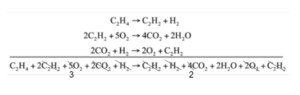Step 2: Calculate the heat of combustion from the reaction enthalpies.

$\Delta H^{\circ} =-1*\Delta H^{\circ}_{r1} + \Delta H^{\circ}_{r2} + \Delta H^{\circ}_{r3}$

$\Delta H^{\circ} = -1* (-174.19 kJ) + (-2511 kJ) + 1013.7 kJ$

$\Delta H^{\circ} = -1323.11 kJ$

# Appendix and Glossary

The appendix introduces some sites and software for finding useful data or perform certain types of calculations. Each session contains a guide to walk through the site or software, and some can be found with a worked example when the site or software is used.

## Appendix: Finding Chemical Pricing Data

Finding this data can be very challenging. Companies typically want to keep pricing information secret (for competition reasons). The best way I have found to search for chemical pricing data is as follows:

1. Go to Texas A&M Chemical Pricing Database
2. Enter a chemical name (i.e. Ethylene) or CAS number (Chemical Abstracts Services; can be found on Wikipedia), chick “EXECUTE QUERY”.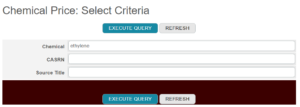3. Find a citation from “ICIS Chemical Business” and note the edition and page number (i.e. 2018, April 6; 19). Make sure the chemical name matches the one you are searching for.4. Go to UBC Library and type in “ICIS Chemical Business” into the search bar and search
5. Click on the source by Factiva, Business Source Ultimate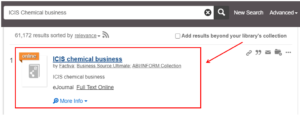6. Click on “Browse Journal”. Find the issue of the journal from the list on the right.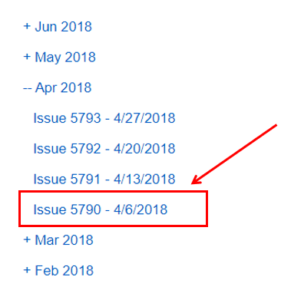7. Find the specific article you found from the Texas A&M Database citation using the dates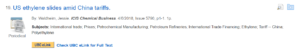8. Once you find your article, click “UBC eLink”. This should take you to a new page where you can access the full text
9. Search through the article for the chemical of interest’s price

This process is tedious and often takes some time, even for people experienced in this process. So don’t get discouraged when looking for this data and feel free to ask questions (through the discussion board, in tutorials or office hours, etc.).

The “Glossary” session in the appendix summarizes the important terms in this course arranged in alphabetical order. You can find a short definition of each term and a link to the section that the term is introduced.